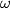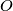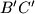### IMO Shortlist 2011 problem G1

Kvaliteta:
Avg: 3,3
Težina:
Avg: 6,3
Let$ABC$ be an acute triangle. Let$\omega$ be a circle whose centre$L$ lies on the side$BC$. Suppose that$\omega$ is tangent to$AB$ at$B'$ and$AC$ at$C'$. Suppose also that the circumcentre$O$ of triangle$ABC$ lies on the shorter arc$B'C'$ of$\omega$. Prove that the circumcircle of$ABC$ and$\omega$ meet at two points.

Proposed by Härmel Nestra, Estonia
Izvor: Međunarodna matematička olimpijada, shortlist 2011